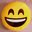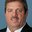Question-and-Answer Resource for the Building Energy Modeling Community
Get started with the Help page

Zone Equipment PTHP rated conditions warning and AHRI test conditon warningI have created a zone equipment PTHP for the first time and applied it to a zone with default values. After running the model I received a few suspicious warnings [below]. My questions are:

1. How can I resolve these warnings?
2. What are E+ conditions for pthp rated heating capacity?

GetDXCoils: Coil:Heating:DX:SingleSpeed="PTHP 1 SPD DX HTG COIL" curve values * ~~~ * ... Heating Capacity Function of Temperature Curve Name = OS:CURVE:BIQUADRATIC 11 output is not equal to 1.0 (+ or - 10%) at rated conditions. Curve output at rated conditions = 0.786

Coil:Heating:DX:SingleSpeed=PTHP 1 SPD DX HTG COIL: Heating Seasonal Performance Factor calculated is not at the AHRI test condition. Review the Standard Ratings calculations in the Engineering Reference for this coil type. Check limits in Total Heating Capacity Function of Temperature Curve, Curve Type = Curve:Biquadratic, Curve Name = OS:CURVE:BIQUADRATIC 11. Check limits in EIR Function of Temperature Curve, Curve Type = Curve:Biquadratic, Curve Name = OS:CURVE:BIQUADRATIC 12

Biquadratic 11 in my model refers to the "Total Heating Capacity Function of Temperature Curve Name" field, while Biquadratic 12 refers to "Energy Input Ratio Function of Temperature Curve Name" field.

To my knowledge I had not changed the values of OS:CURVE:BIQUADRATIC 11 - they are as follows: - Coefficient1 Constant = 0.7587 - Coefficient2(x); Coefficient3(x2) = 0 - Coefficient4y = 0.027626 - Coefficient5y2 = 0.0001487 - Minimum Value of x, y = 0 - Maximum Value of x,y = 1

To my knowledge I had not changed the values of OS:CURVE:BIQUADRATIC 12 - they are as follows: - Coefficient1 Constant = 1.19248 - Coefficient2(x) = -0.03004 - Coefficient3(x2) = 0.00103745 - Coefficient4y = 0 - Coefficient5y2 = 0 - Min/Max Value of x = {-20,20} - Min/Max Value of y = {0,1}

I've scanned around pg 1785 of E+ IO Reference Manual and could not find a definition of rated conditions. I learned from IO reference the Total heating Capacity Function of Temperature curve is normalized to 1, with x and y being indoor and outdoor temperatures.

I looked at a PTAC example file with same curve type and the x and y values were greater than 1. To mimic this, I tried increasing the max/min 'y' value to a more reasonable temperature number like 20/0 degC for CURVE 11 and that has resolved the first error. I find it a bit suspicious that the default max value would be 1 if it were incorrect so I'm a bit confused.

1. Did I accidentally change this, or is there some reason why the max 'y' value defaults to 1 for curve 11?
edit retag close merge delete

Sort by » oldest newest most votedThe AHRI rating conditions for DX cooling coils is indoor wet-bulb temperature = 19.44 C (67 F) and outdoor dry-bulb temperature = 35 C (95 F). If you use these temperatures in your performance curve equation you should see where the 0.786 is coming from. At this condition the program expects a value of 1.0 +- 10%. Performance curves will typically be normalized to 1 at this rating point.

The next warning Heating Seasonal Performance Factor calculated is not at the AHRI test condition is telling you that the performance curves entered do not offer the performance at the conditions used to evaluate HSPF. This is due to the performance curve's min/max X and Y not including the indoor wet-bulb and outdoor dry-bulb temperatures used during this rating procedure calculation. See the Engineering Reference section Single-Speed DX Heating Coil Standard Ratings for more information.

more

I will look up the equivalent AHRI rating for a dx heating coil and set limits based on that. Thanks for the help.